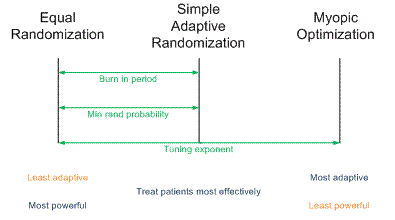# Three ways of tuning an adaptively randomized trial

Yesterday I gave a presentation on designing clinical trials using adaptive randomization software developed at M. D. Anderson Cancer Center. The heart of the presentation is summarized in the following diagram.(A slightly larger and clearer version if the diagram is available here.)

Traditional randomized trials use equal randomization (ER). In a two-arm trial, each treatment is given with probability 1/2. Simple adaptive randomization (SAR) calculates the probability that a treatment is the better treatment given the data seen so far and randomizes to that treatment with that probability. For example, if it looks like there’s an 80% chance that Treatment B is better, patients will be randomized to Treatment B with probability 0.80. Myopic optimization (MO) gives each patient what appears to be the best treatment given the available data with no randomization.

Myopic optimization is ethically appealing, but has terrible statistical properties. Equal randomization has good statistical properties, but will put the same number of patients on each treatment, regardless of the evidence that one treatment is better. Simple adaptive randomization is a compromise position, retaining much of the power of equal randomization while also treating more patients on the better treatment on average.

The adaptive randomization software provides three ways of compromising between the operating characteristics ER and SAR.

1. Begin the trial with a burn-in period of equal randomization followed by simple adaptive randomization.
2. Use simple adaptive randomization, except if the randomization probability drops below a certain threshold, substitute that minimum value.
3. Raise the simple adaptive randomization probability to a power between 0 and 1 to obtain a new randomization probability.

Each of these three approaches reduces to ER at one extreme and SAR at the other. In between the extremes, each produces a design with operating characteristics somewhere between those of ER and SAR.

In the first approach, if the burn-in period is the entire trial, you simply have an ER trial. If there is no burn-in period, you have an SAR trial. In between you could have a burn-in period equal to some percentage of the total trial between 0 and 100%. A burn-in period of 20% is typical.

In the second approach, you could specify the minimum randomization probability as 0.5, negating the adaptive randomization and yielding ER. At the other extreme, you could set the minimum randomization probability to 0, yielding SAR. In between you could specify some non-zero randomization probability such as 0.10.

In the third approach, a power of zero yields ER. A power of 1 yields SAR. Unlike the other two approaches, this approach could yield designs approaching MO by using powers larger than 1. This is the most general approach since it can produce a continuum of designs with characteristics ranging from ER to MO. For more on this approach, see Understanding the exponential tuning parameter in adaptively randomized trials.

So with three methods to choose from, which one do you use? I did some simulations to address this question. I expected that all three methods would perform about the same. However, this is not what I found. To read more, see Comparing methods of tuning adaptive randomized trials.

Update: The ideas in this post and the technical report mentioned above have been further developed in this paper.